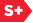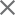### Proceedings Paper

Tensor representation of color images and fast 2D quaternion discrete Fourier transform
Format Member Price Non-Member Price
PDF \$17.00 \$21.00

Paper Abstract

In this paper, a general, efficient, split algorithm to compute the two-dimensional quaternion discrete Fourier transform (2-D QDFT), by using the special partitioning in the frequency domain, is introduced. The partition determines an effective transformation, or color image representation in the form of 1-D quaternion signals which allow for splitting the N × M-point 2-D QDFT into a set of 1-D QDFTs. Comparative estimates revealing the efficiency of the proposed algorithms with respect to the known ones are given. In particular, a proposed method of calculating the 2r × 2r -point 2-D QDFT uses 18N2 less multiplications than the well-known column-row method and method of calculation based on the symplectic decomposition. The proposed algorithm is simple to apply and design, which makes it very practical in color image processing in the frequency domain.

Paper Details

Date Published: 16 March 2015
PDF: 12 pages
Proc. SPIE 9399, Image Processing: Algorithms and Systems XIII, 93990N (16 March 2015); doi: 10.1117/12.2083199
Show Author Affiliations
Artyom M. Grigoryan, The Univ. of Texas at San Antonio (United States)
Sos S. Agaian, The Univ. of Texas at San Antonio (United States)

Published in SPIE Proceedings Vol. 9399:
Image Processing: Algorithms and Systems XIII
Karen O. Egiazarian; Sos S. Agaian; Atanas P. Gotchev, Editor(s)